## Math with LEGO Lee De Cola`ldecola.net/projects/LEGO`

### Bar plots Sine Circles and radii Data visualization Proofs without words Pi

This webpage illustrates some of the patterns I have made with LEGO. If you define mathematics as "the science of patterns" then LEGO are a tactile/visual computer to explore mathematics. Most of these experiments are in 2 dimensions, which seems quite enough space to get started!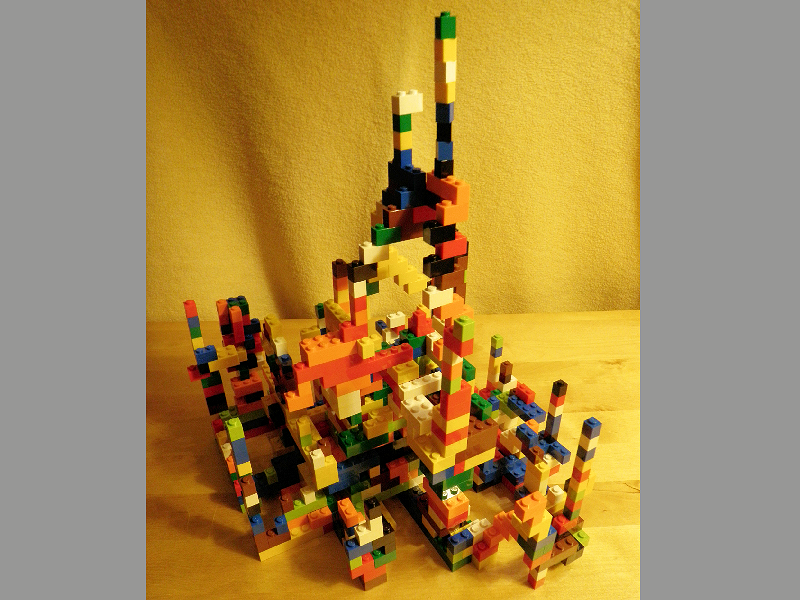### Pile

When I opened the box I started to connect all of the 650 bricks in set #6177 in no particular order. As someone with a knowledge of discrete mathematics, I soon recognized that any connected "pile" of a finite number of bricks was one arrangement from a finite number of such arrangements, even though that number would be extremely large (probably beyond the number of particles in the universe).

## Bar plots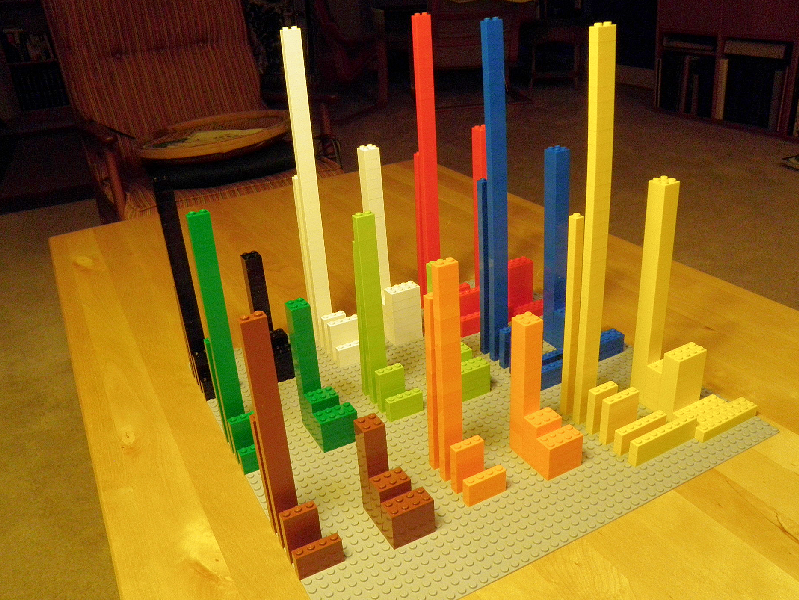### 3D bar plot I

All of the LEGO in the set are arranged by length × width × color. A true histogram uses the same symbology (usually same-width rectangles) to represent frequencies within same-with intervals, but this is a nice example of how objects can be used to represent their own frequencies.

This is an oblique view.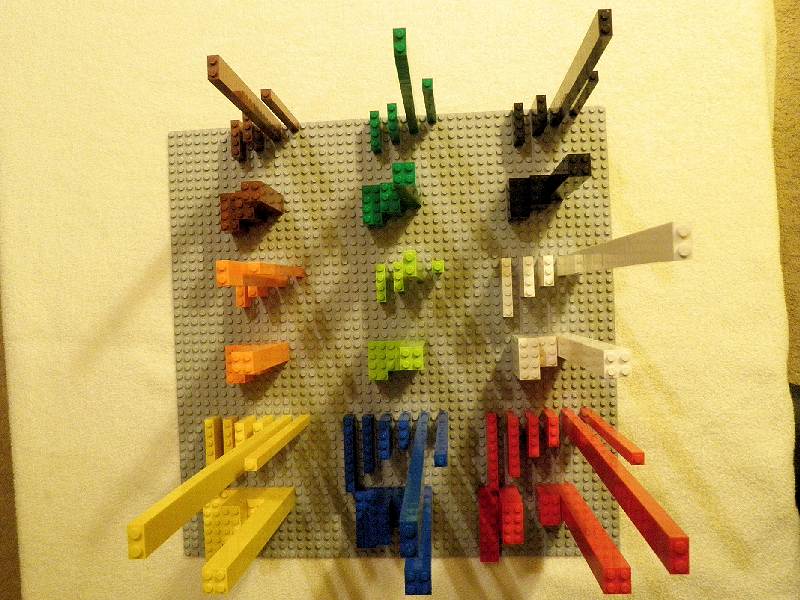### 3D bar plot II

All of the 650 bricks in the #6177 LEGO set are arranged by length × width × color.

This is a plan view.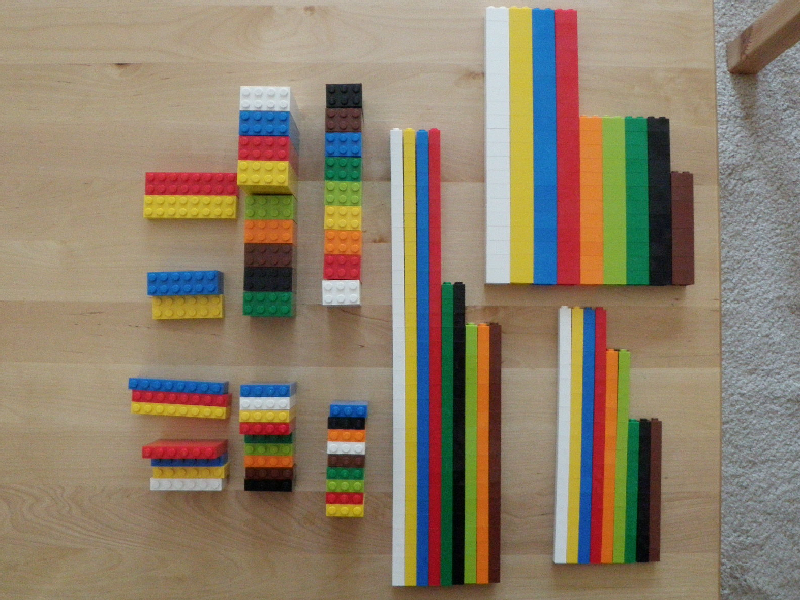### Grouped I

Here are all the bricks laid out by color × frequency × length × width. This is a useful way to organize the bricks out of the box to see exactly what you have, and it’s a natural way to illustrate hierarchical classification: dim1 × dim2 × dim3, with the first dimension changing most rapidly, etc.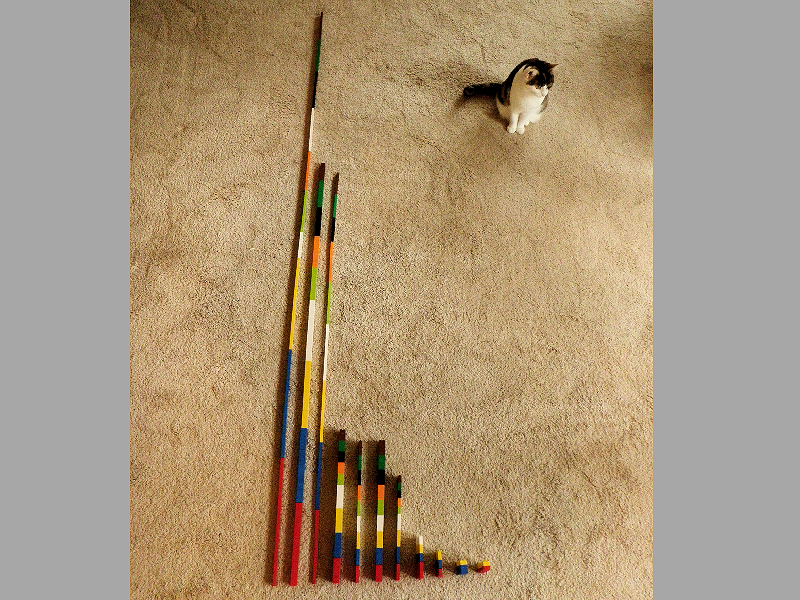### Grouped II

Another way of arranging all the bricks that requires much more space. The cat is for scale.

## Sine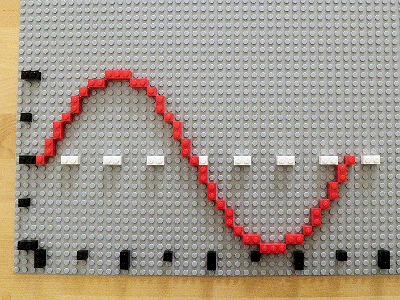### Sine

This sine curve was my first attempt at illustrating mathematics with LEGO. At first I merely computed the rounded values of the sine function at every 10 degrees (spanning 36 units) but this left gaps in the function, which I filled in by computing the inverse, arcsine() function.

The exercise helps you to understand the problem of "aliasing" in computer graphics.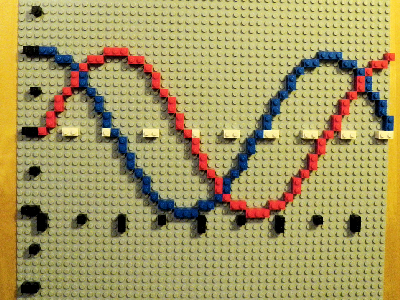### Sine and cosine

As you add bricks to the sine representation you acquire a nice sense of the rhythms of the pattern: first you realize that the arrangement repeats every cycle, then (in a negative direction) every half-cycle, then as a mirror image every quarter-cycle.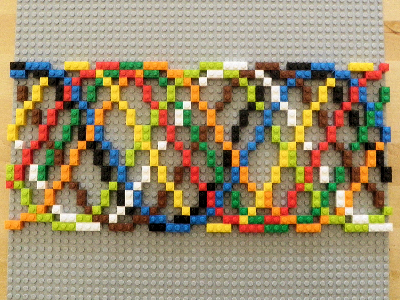### Multi-phase

I continued with the sine curve in multiple phases, trying to fill up the space. I think I ran out of enough distinct colors, but I’m sure that finishing this − and extending it in the horizontal direction − would make a pleasing pattern. There are only 9 colors in the set I used, but I think there are a couple of dozen more.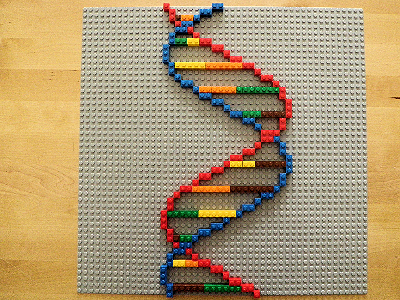### Double helix

A variation on the sine function is the addition of another sine with 2-color connectors every 4 units. The use of only 4 colors for the connectors represents the 4 amino acids.

## Circles and radii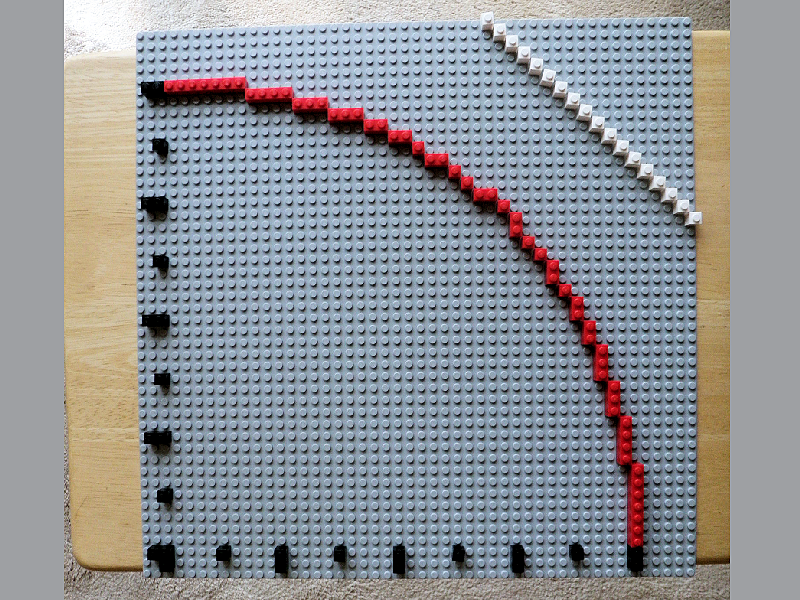### A circle

A circle is defined by r2 = x2 + y2 or y = sqrt(r2x2).

Here’s circle with radius 40. As with the sine curve, you have to compute the value of the function until its derivative is < −1 (in brick-space), then you need the inverse.

Part of a circle of infinite radius is shown in white.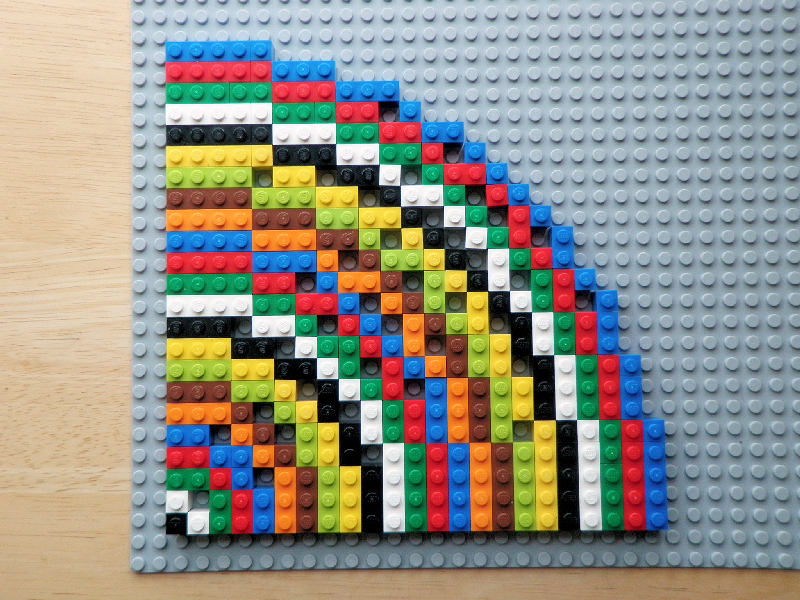### Circles I

To plot circles of radius = 0, 1, ⋯., 22 (when I ran out of orange), I wrote an R script that did the following:
• compute the rounded values of y for r = 1, 2, ⋯, n / √2 because you only need values up to the line of equality y = x,
• determine the run length encodings for each r,
• print out only lengths of each run.
Here are a few results:

```  18: 5 3 2 1 1 1
19: 5 3 2 1 2 1
20: 5 3 2 2 1 1 1
21: 5 3 2 2 1 2 1
22: 5 3 3 1 2 1 1```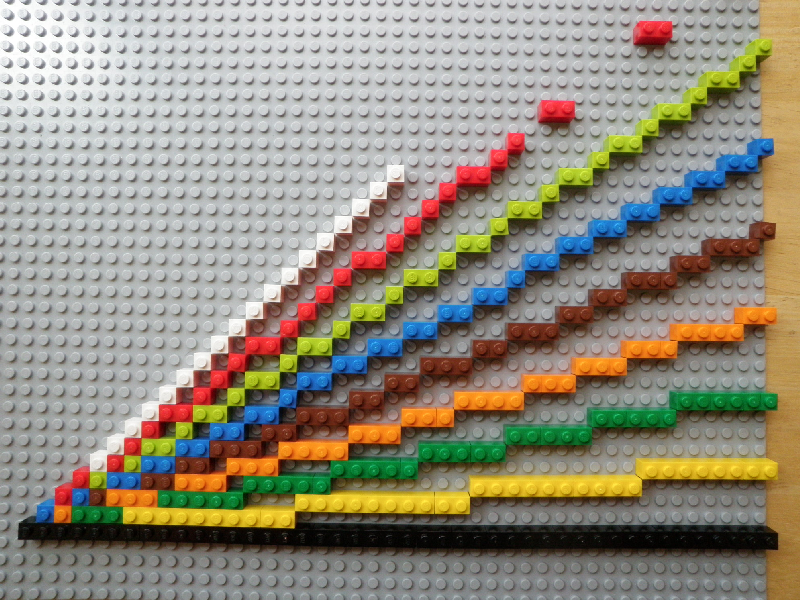### Radii

For each angle θ in the set {i × π/32 | i = 0, 1, ⋯, 8} compute for each x = 0, 1, ⋯, 48 the value y = round(x tan(θ)). (The gray base board is 48 bricks wide.)

These brick lengths can also be summarized with run length encoding. Here’s the result for angle #6 (blue):

```  COUNT: 1 7 1 6 1 7 1 2
BRICK: 1 2 1 2 1 2 1 2```

Start with a 1-brick, then seven 2-bricks moving up each time, then one 1-brick, six 2-bricks, etc. For angles #8 and #9 I ran out of 1×1-bricks. And you might think from examining the ‘lime’ bricks that tan(3/16 π) = 2/3, but at x = 112 units you’d encounter a break in the 1-2 pattern.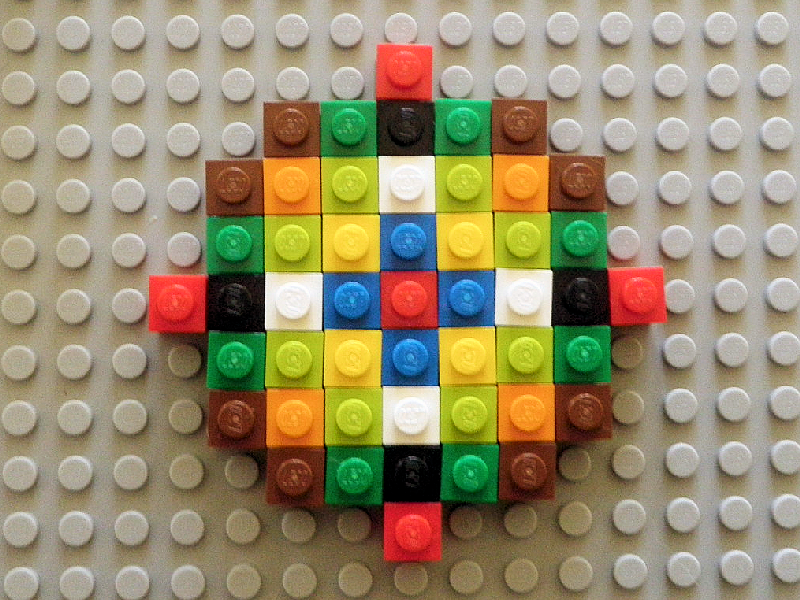### Circles II

Cells are at increasing distances from a central cell, here each distance is shown with a different color. I should add to this model, which I think requires only 1 × 1 bricks.

## Data visualization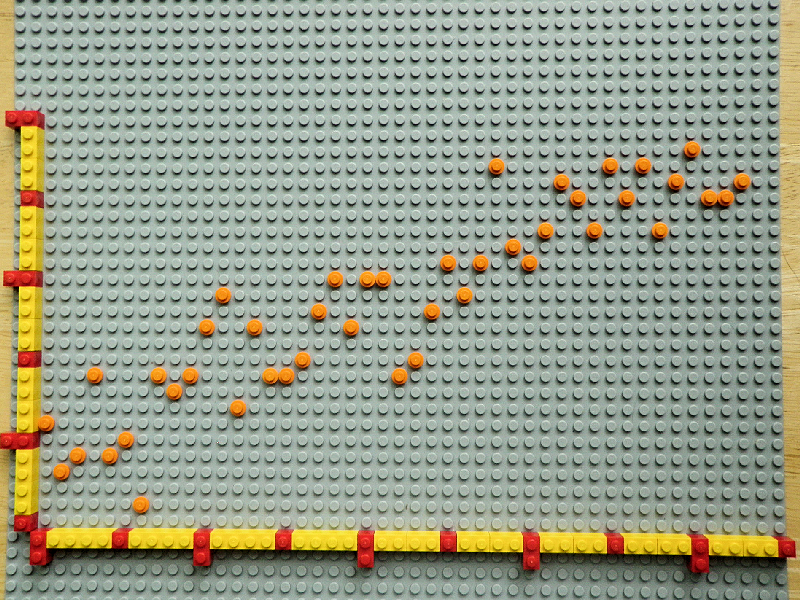### Time series

This visualization plots yearly global temperature anomalies from NASA. The horizontal axis spans the years 1970 to 2015 with 1×1 and 1×2 bricks marking 5 and 10 years respectively. The vertical axis spans -0.25 to 1.00 °C with bricks marking 0.25 °C increments. The overall trend is upward, but with an apparent recent leveling off. Nevertheless a regression fit to the data suggest a .02 °C/year increase, or over 3 degrees °F per century.

The design is somewhat busy; the ‘buttons’ work well, but the points should be of a greater contrast, and the axes less.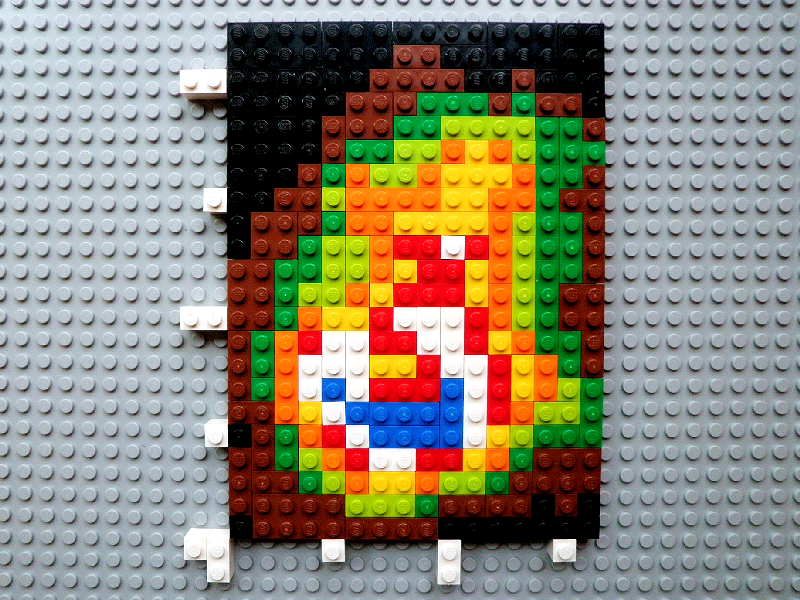### A 22 × 16 grid

This simple ‘map’ was derived from the volcano (Maunga Whau, New Zealand) digital elevation data that comes with the R language (2014). I sampled every 4 rows and columns (so the unit brick = about 40 meters) and collapsed the elevation values to 9 classes. The colors were selected from those available to suggest an island with a brown shoreline, lowland vegetation, arid uplands, and a lake surrounded by snow.

Spatial autocorrelation gives the image a sense of being about something real; to me the image could also be a false-color micrograph of a bacteria or perhaps a supernova⋯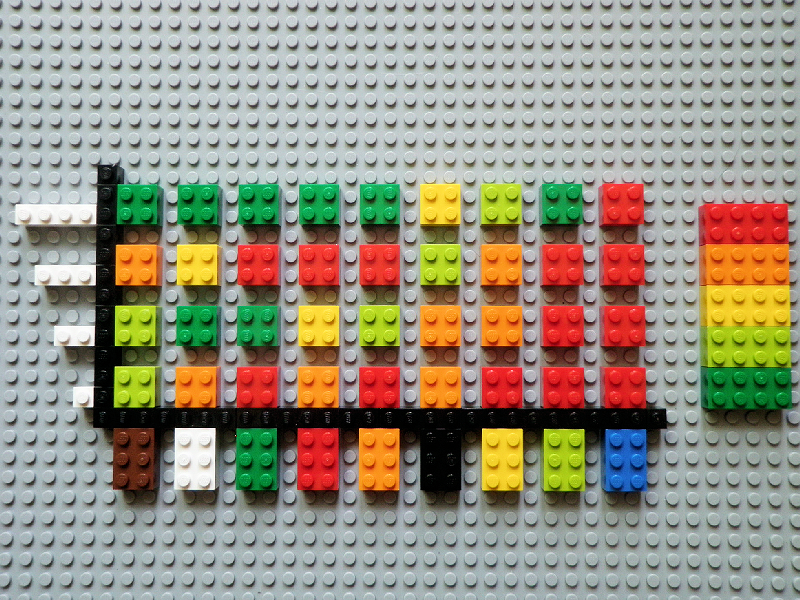### Cracking problem

During Feb-Apr 2014 while I developed the various patterns shown above I began to notice cracks in the bricks. Overall, 257 or 40% of the bricks from the 650 in the set #6177 that I originally purchased showed some signs of cracking on at least one (and occasionally up to 4) sides. All but 2 of the cracks appeared in the width-1 bricks, for which a detailed analysis of the incidence of failure is summarized here with representation in which colors and lengths of width-1 bricks showed cracks. The crosstab shows the 4 lengths by the 9 colors and the 5 colors on the right represent incidence with breaks at 20, 40, 60, and 80%. The image shows that the 1 × 1 bricks fail most frequently, as do the blue and lime, but of course this is not a scientifically, controlled experiment.

Seven types of brick color × length types show cracking in all the items of that class. Although I was given a new set of bricks, I have not yet received a satisfactory answer from Lego.

## Proofs without words

I was introduced to Nelsen (1993) by Lynn Salvo of MathCamp. It’s a wealth of ideas about how to convey mathematical ideas using a purely visual language. Unit Lego bricks, their grid organization, and a range of colors are all you need to get started.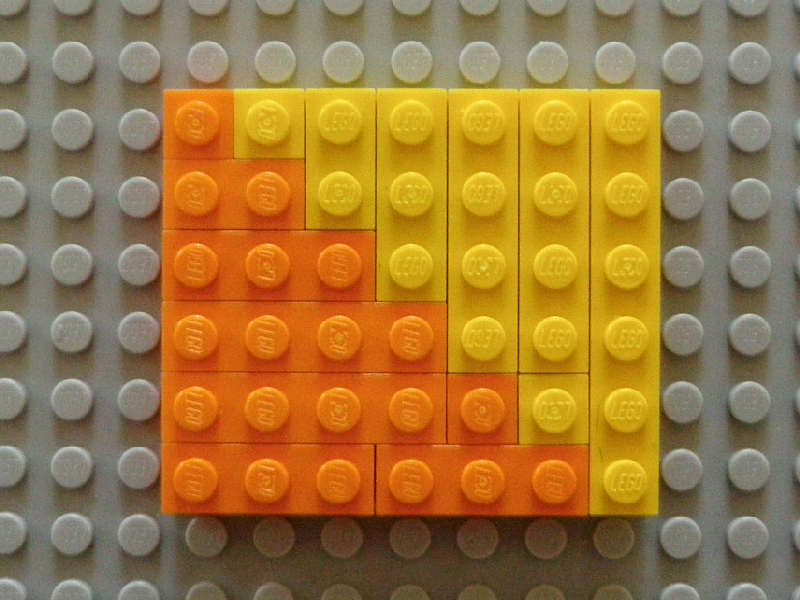### Sums of integers I

This exhibit is step 6 in the theorem that
1 + 2 + ⋯ + n = 1/2 n (n + 1).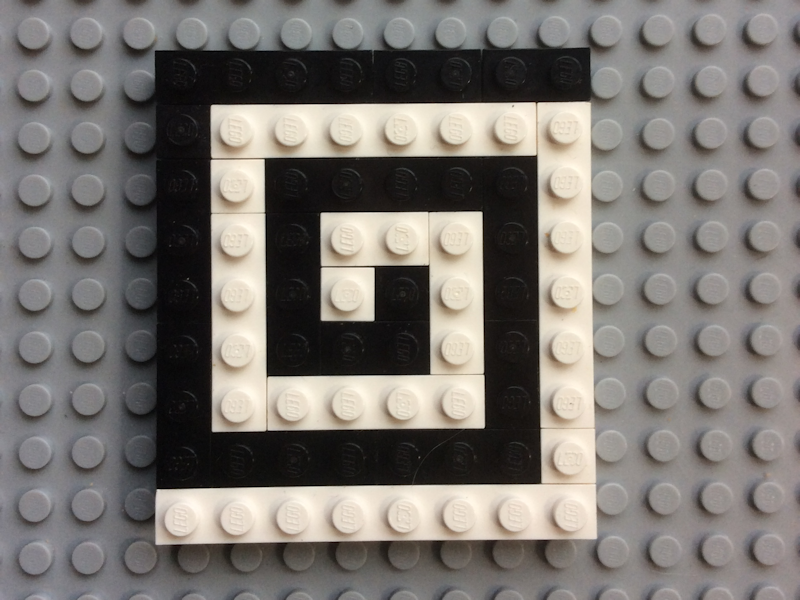### Sums of integers III

Although it’s not in Helsen (1993) this exhibit also illustrates step 8 of the above theorem.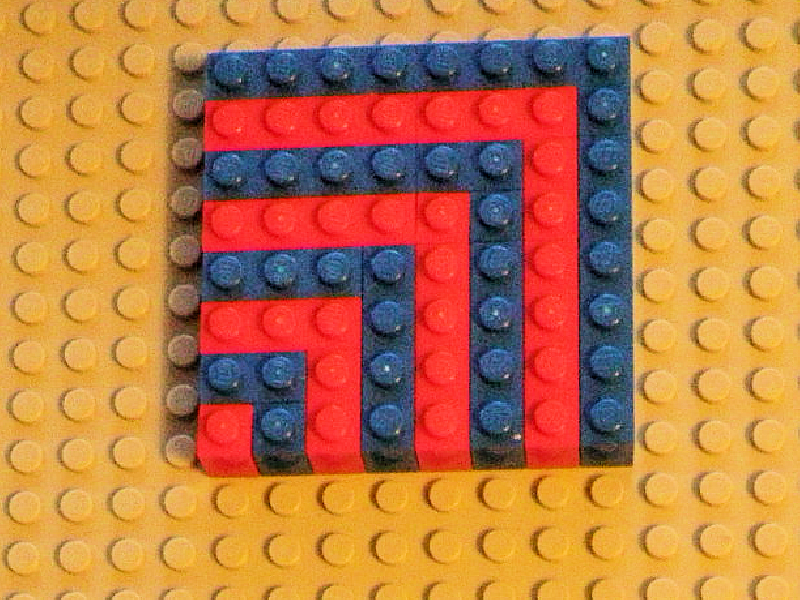### Sums of odd integers I

This exhibit shows step 8 of the illustration that
1 + 3 + ⋯ + (2n − 1) = n2.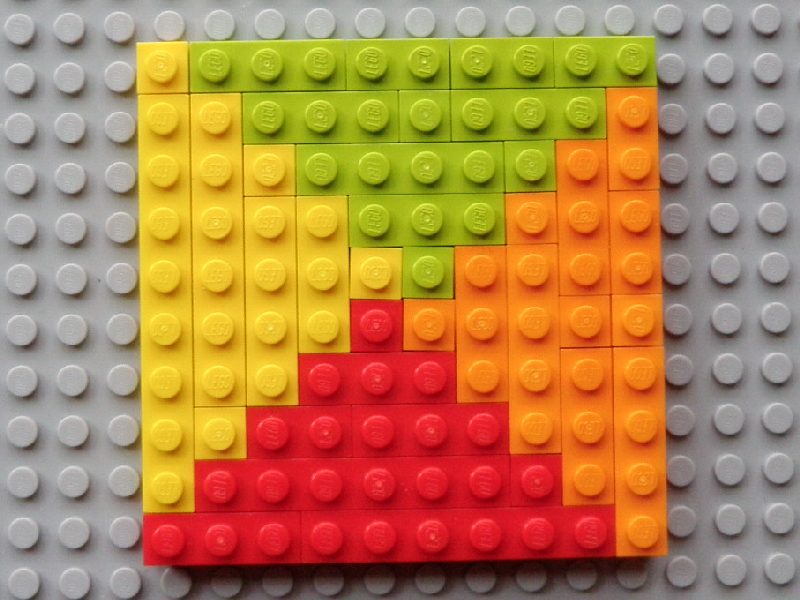### Sums of odd integers II

Step 5 of the illustration that
1 + 3 + ⋯ + (2n − 1) = 1/4 (2n)2 = n2.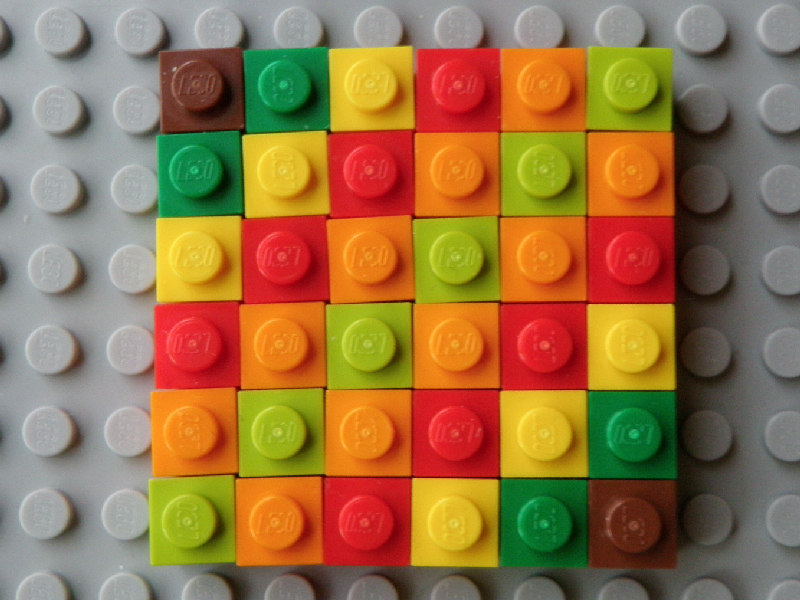### Squares and sums of integers

Step 6 of the illustration that
1 + 2 + ⋯ + (n − 1) + n + (n − 1) + ⋯ + 2 + 1 = n2.

## Pi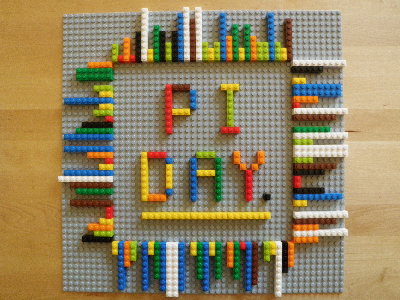### Digits of pi

For Pi Day my wife Freya and I arrayed 120 digits of pi using a different color for each digit. The design also illustrates how to make letters on a 5 by 8 grid.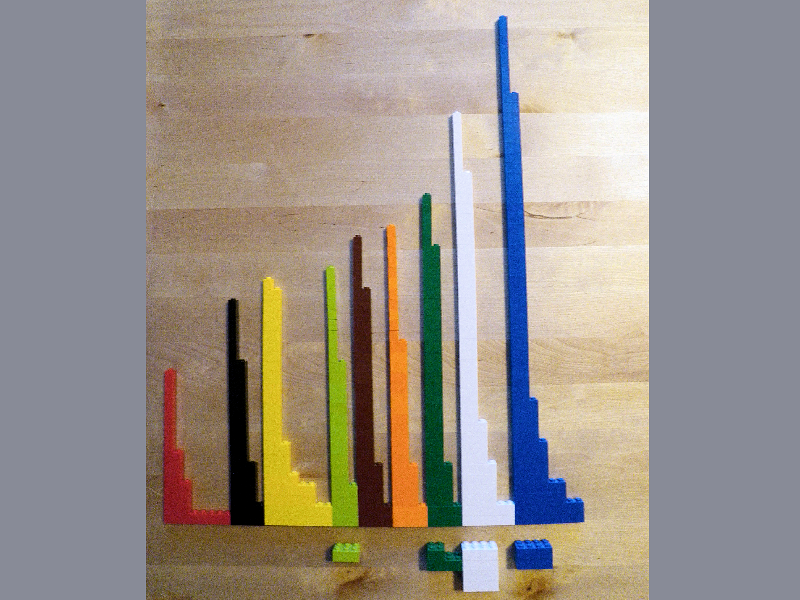### Materials for pi

Here are all the bricks for the previous exhibit (including the title) arranged by color length × color × width. This exhibit illustrates the process either of categorizing the objects that make up one pattern, or of assembling the materials to create a pattern.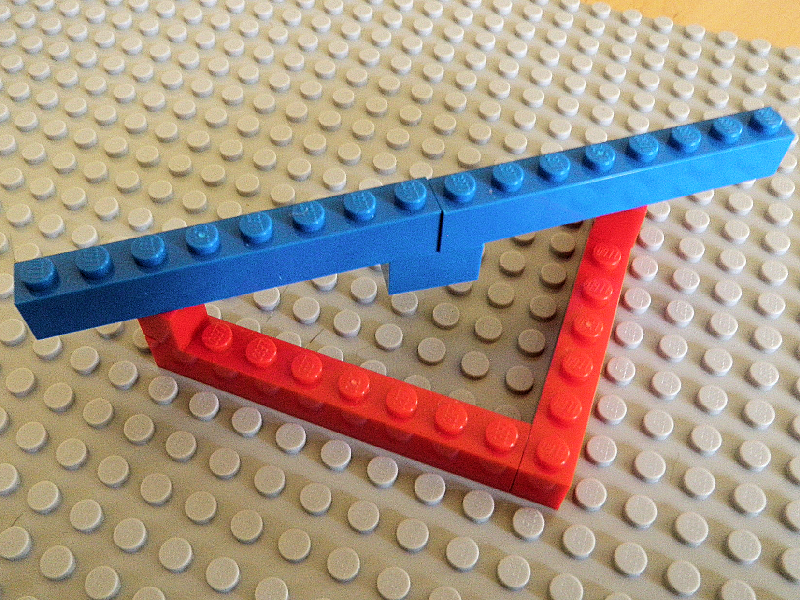### A Pythagorean triangle

The 3-4-5 Pythagorean triangle is here doubled. You can’t do this in the ‘two dimensions’ of a single brick layer.

## References

Nelsen, Roger B (1993) Proofs without words: exercises in visual thinking. Washington, D.C., The Mathematical Association of America.

R Core Team (2014) R: A language and environment for statistical computing. R Foundation for Statistical Computing, Vienna, Austria. www.R-project.org